## A negative point charge q1 = 25 nC is located on the y axis at y = 0 and a positive point charge q2 = 10 nC is located at y =14 cm. Find the

Question

A negative point charge q1 = 25 nC is located on the y axis at y = 0 and a positive point charge q2 = 10 nC is located at y =14 cm. Find the y coordinate of the points where the net electric potential due to these two charges is zero.

in progress 0
6 months 2021-07-12T12:10:04+00:00 1 Answers 9 views 0

## Answers ( )

y = 0.1 m

Explanation:

The electrical power for point loads is

V =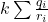k Sum qi / ri

in this case

V = k (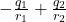)

indicate that V = 0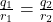r₂ =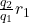the distance r1 is

r₁ = y -0

the distance r2

r₂ = 0.14 -y

we substitute

0.14 – y =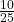y

y (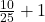) = 0.14

y 1.4 = 0.14

y = 0.14 / 1.4

y = 0.1 m